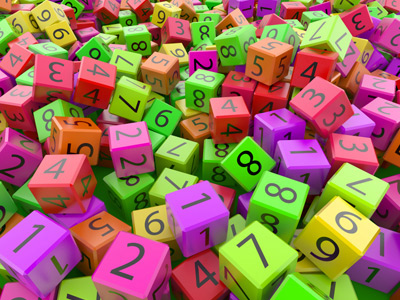Can you put all these numbers in the right order?

# Compare and Order Numbers 2 (Medium)

In maths, there are many different words and symbols which you need to familiarise yourself with. The better you know them, and what they mean, the easier you will find maths when you get to secondary school. That’s where this quiz comes in!

The more you are exposed to the various mathematical terms, the more confident you will become – until you find maths is a breeze! But be careful - remember to read the questions carefully before choosing your answer. Even the very best mathematicians must be cautious if they don't want to fall foul of silly mistakes.

Good luck!

1.
Which one of the following expressions is not correct?
17 < 17
17 > -17
-17 < 17
17 > 0
17 is equal to 17, not less than. The correct symbol would be ≥, because 17 ≥ 17. The > and < symbols DO NOT include 'equal to' - DON'T forget this
2.
Which one of the expressions below is correct?
3 ≤ 9 ≤ 6
9 ≤ 6 ≤ 3
3 ≤ 6 ≤ 9
9 ≤ 3 ≤ 6
3 is less than 6 which is less than 9. The numbers MUST be in the correct order of size (magnitude) for this to work. In the first choice, 3 ≤ 9 BUT 9 IS NOT ≤ 6
3.
Which one of the following expressions is not correct?
6 ≤ 6
-6 ≤ 6
0 ≤ 6
6 ≤ 0
Of course, 6 is greater than 0, not less than!
4.
If a piece of wire is > 2 m and ≤ 7 m, what are the shortest and longest possible lengths of the wire, in whole metres?
The shortest length = 2 m and longest length = 7 m
The shortest length = 3 m and longest length = 6 m
The shortest length = 3 m and longest length = 7 m
The shortest length = 2 m and longest length = 6 m
The shortest length is more than 2 m, but the longest length can be up to 7 m. This is what ≤ 7 means: less than OR equal to 7.
5.
Which number lies mid-way between 1,973 and 3,845?
2,894
2,909
2,944
2,974
Here's how you solve problems like this: add both numbers together THEN divide by two: (1,973 + 3,845) = 5,818. So, 5,818 ÷ 2 = 2,909
6.
Which one of the following expressions is not correct?
17 ≥ 17
17 ≥ 20
17 ≥ 0
17 ≥ -17
20 is greater than 17.
7.
What is the smallest number that can be formed using the digits 4, 6, 8, 9?
4,689
4,869
4,698
4,986
Write the digits in descending order to find the BIGGEST number. Write the digits in ascending order to find the SMALLEST number
8.
Which one of the expressions below is correct?
16 ≥ 16 ≥ 20
10 ≥ 16 ≥ 10
10 ≥ 10 ≥ 16
16 ≥ 10 ≥ 10
16 is greater than 10 which is equal to 10. The numbers MUST be in the correct order of size (magnitude) for this to work. In the first choice, 16 ≥ 16 BUT 16 IS NOT ≥ 20
9.
Stephen is > 140 cm tall. Angela is ≥ 140 cm tall. Which one of the statements below is definitely true?
Angela and Stephen are the same size
Stephen is taller than Angela
Angela might be shorter than Stephen
Angela is taller than Stephen
Angela could be any height from 140 cm upwards. Stephen could be any size taller than 140 cm - so Stephen could be the smallest (e.g. Stephen is 144 cm and Angela is 150 cm). The ≥ symbol allows this possibility BUT the > symbol does not
10.
Which one of the numbers below is < -324?
-323
-325
-324
0
As you move from zero to the left along the number line, the values of the numbers are negative and they get less and less: so -325 < -324 because it is more to the left
Author:  Frank Evans